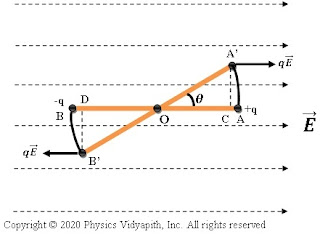### Work done by a rotating electric dipole in uniform electric field

Derivation:

Let us consider, An electric dipole AB, made up of two charges $+q$ and $-q$, is placed at a very small distance $2l$ in a uniform electric field $\overrightarrow{E}$. If dipole $AB$ rotates at angle $θ$ from its equilibrium position. If $A'$ and $B'$ are the new position of a dipole in the electric field. Then force on $+q$ charge particle due to electric field→

$\overrightarrow{F_{+q}}=q\overrightarrow{E}\qquad (1)$

Then force on $-q$ charge particle due to electric field→

$\overrightarrow{F_{-q}}=q\overrightarrow{E}\qquad(2)$Work done by rotating an electric dipole
So work done by a force on $+q$ charge particle to bring from position $A$ to position $A'$→

$\overrightarrow{W_{+q}}=\overrightarrow{F_{+q}}·\overrightarrow{AC}$

$\overrightarrow{W_{+q}}=q\overrightarrow{E}· \overrightarrow{AC}\qquad(3)$

Similarly, work done by the force on $-q$ charge particle to bring from position $B$ to position $B'$→

$\overrightarrow{W_{-q}}=\overrightarrow{F_{-q}}·\overrightarrow{BD}$

$\overrightarrow{W_{-q}}=q\overrightarrow{E}· \overrightarrow{BD}\qquad (4)$

So the total work is done by the force on the dipole→

$\overrightarrow{W}=\overrightarrow{W_{+q}}\:+\:\overrightarrow{W_{-q}}$

$\overrightarrow{W}=q\overrightarrow{E}·(\overrightarrow{AC}+\overrightarrow{BD})\qquad (5)$

From the figure, There is symmetry so

$\overrightarrow{AC}=\overrightarrow{BD}$

So from equation $(5)$

$\overrightarrow{W}=q\overrightarrow{E}·(2\overrightarrow{AC})$

$\overrightarrow{W}=2q\overrightarrow{E}(\overrightarrow{AO}-\overrightarrow{CO})\qquad (6)$

From figure→

$\left | \overrightarrow{AO} \right |=l$

$\left | \overrightarrow{CO} \right |=l\:cos \theta$

Now substitute the values in equation (6). So equation (6) can be written as in magnitude form →

$W=2qE(l-l\:cos\theta )$

$W=2qEl(1-cos\theta )$

 $W=pE(1-cos\theta )$

The above expression shows that work is done on a rotating electric dipole in a uniform electric field.

Case (i):

If $\theta=0^{\circ}$, Then work done will be minimum

 $W_{min}=0$

Case (ii):

If $\theta=90^{\circ}$, Then work done

 $W=pE$

Case (iii):

If $\theta=180^{\circ}$, Then work done will be maximum

 $W_{max}=2pE$